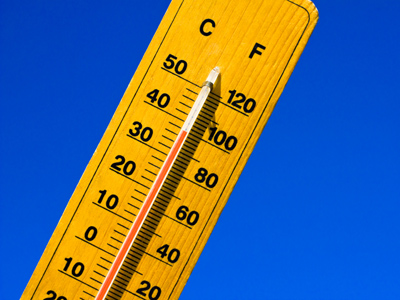Temperatures are measured in oC (Celsius) or sometimes in oF (Fahrenheit).

# Year 2 Measurements - Units

This quiz addresses the requirements of the National Curriculum KS1 Maths and Numeracy for children aged 6 and 7 in year 2. Specifically this quiz is aimed at the section dealing with choosing and using appropriate standard units to estimate and measure.

Children in Year 1 are exposed to and expected to use the appropriate standard units of measurement for height (cm/m), weight (g/kg), temperature (oC) and capacity (ml/l). This quiz will help them to become familiar with the appropriate units to use for each measurement.

To see a larger image, click on the picture.
Question 1
Which unit would we use to measure the length of the book?
Centimetres
Metres
Millilitres
Grams
The book is about 40cm long
Question 2
Which unit would we use to measure the amount of water in the bath?
Litres
Centimetres
Grams
Kilometres
Litres are useful for larger amounts of liquid
Question 3
Which unit would we use to measure the temperature on the island?
Litres
Centimetres
oC
Grams
Temperatures are measured in degrees
Question 4
Which unit would we use to measure the weight of the cow?
Kilograms
Centimetres
Litres
Metres
A cow weighs more than 500kg!
Question 5
Which unit would we use to measure the length of the caterpillar?
Metres
Centimetres
Litres
Grams
This caterpillar is about 7 or 8 centimetres long
Question 6
Which unit would we use to measure how much juice is in the glass?
Litres
Millilitres
Centimetres
Grams
The juice is less than a litre so we would use millilitres
Question 7
Which unit would we use to measure the height of a tall building?
Kilograms
Millimetres
Centimetres
Metres
Some tall buildings can be hundreds of metres tall!
Question 8
What units could we use to measure his height?
Kilometres and metres
Litres and millilitres
Kilograms and grams
Metres and centimetres
He might be just over a metre tall so we'd need to use centimetres too
Question 9
Which unit would we use to measure how much water the boy will put in the pool?
Litres
Grams
Metres
Millilitres
There will be about 100 litres in the pool
Question 10
Which unit would we use to measure one of the tomatoes' weight?
Kilograms
Grams
Millimetres
Litres
Kilograms wouldn't be any use as the tomato wouldn't be heavy enough
Author:  Angela Smith